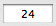﻿ Recognizing the patterns x^n and (-x)^n

#Recognizing the patterns $\,x^n\,$ and $\,(-x)^n$

You may want to explore these related concepts first:

## Examples

In the exercise, you will be filling in the blanks:

 The expression $\,(-y)^{24}\,$ is of the form $\,(-x)^n\,$ where $\,x\,$ isand $\,n\,$ isNote that in the pattern $\,(-x)^n\,,$ the variable ‘$\,x\,$’ represents whatever comes after the minus sign, and the variable ‘$\,n\,$’ represents the exponent.

The expression $\,(-3x)^{13}\,$ is of the form $\,(-x)^n\,$ where $\,x\,$ isand $\,n\,$ is.

Try not to be confused by the appearance of the variable ‘$\,x\,$’ in two places! Again, we're matching something to the pattern $\,(-x)^n\,,$ where ‘$\,x\,$’ represents whatever comes after the minus sign. What comes after the minus sign in $\,(-3x)^{13}\,$?   Answer: $\,3x\,$

The expression $\,(-2x)^{7}\,$ is of the form $\,x^n\,$ where $\,x\,$ isand $\,n\,$ is.

Here, we're matching something to the pattern $\,x^n\,,$ so ‘$\,x\,$’ represents the entire base.

## Practice

 The expression is of the form where $\,x\,$ is and $\,n\,$ is